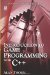# 1.7 Discrete and Continuous Sets

## 1.7 Discrete and Continuous Sets

The numerals and quantities seen so far have been countable sets belonging to discrete mathematics. This means we have been counting items as physically indivisible units; three apples, two chairs, five people, and so on. All of these items are single, discrete quantities that can be counted. They are each one unit and it is not possible to break them into smaller parts such as half, because if any one of them were divided into smaller parts-like half a person-they would no longer be a single, complete unit that we can count in the same way as before. To illustrate the point more clearly, consider the line below.Figure 1.2

In the figure there is one line-the number of lines presented in the figure can be counted. But is it possible to count the actual length of the line in the same way, or the partial length of some subset of the line? A more detailed inspection of the line will reveal that we are dealing with a different kind of quantity, one that is not counted but instead is measured. The length of the line is not composed of discrete and countable units, but it is said to be continuous. It is possible to divide the line into several parts and each part will still be a line, and each of them is still parts of a whole, longer line. The line could be cut into halves, tenths, hundredths, thousandths, and even more divisions, and no matter how small the divisions were-no matter how many smaller components the line were broken into-it would still have some measurable distance. This is the difference between discrete and continuous data.

The ways in which discrete units are counted has already been presented, but the method by which continuous data is expressed varies. This chapter shall examine the method called fractions. As can be seen, fractions are used not for counting but for measuring.Introduction to Game Programming with C++ (Wordware Game Developers Library)
ISBN: 1598220322
EAN: 2147483647
Year: 2007
Pages: 225
Authors: Alan Thorn

Similar book on Amazon